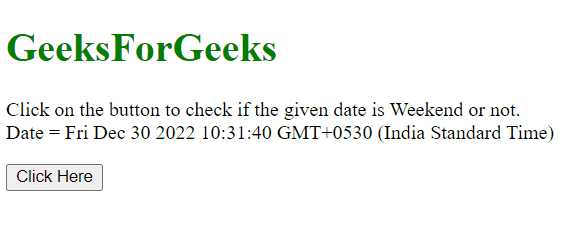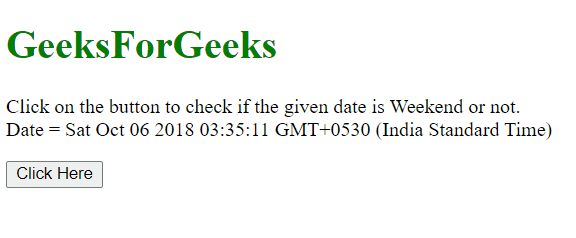GFG App
Open AppBrowser
Continue

# How to check if the given date is weekend ?

Given a date and the task is to determine if the given date is a weekend (In this case we are considering Saturday as the weekend. There are two methods to solve this problem which are discussed below:

Approach 1:

• Use .getDay() method on the Date object to get the day.
• Check if it is 6 or 0 (6 denotes Saturday and 0 denotes Sunday).

Example: This example implements the above approach.

## html

 ` ` `<``html``> ` ` `  `<``head``> ` `    ``<``title``> ` `        ``How to check if the given ` `        ``date is weekend ? ` `    `` ` ` ` ` `  `<``body``> ` `    ``<``h1` `style``=``"color:green;"``> ` `        ``GeeksForGeeks ` `    `` ` ` `  `    ``<``p` `id``=``"GFG_UP"``> ` `    `` ` ` `  `    ``<``button` `onclick``=``"gfg_Run()"``> ` `        ``Click Here ` `    `` ` ` `  `    ``<``p` `id``=``"GFG_DOWN"``> ` `    `` ` ` `  `    ``<``script``> ` `        ``var el_up = document.getElementById("GFG_UP"); ` `        ``var el_down = document.getElementById("GFG_DOWN"); ` `        ``var date = new Date(); ` `         `  `        ``el_up.innerHTML = "Click on the button to " ` `                ``+ "check if the given date is Weekend" ` `                ``+ " or not.<``br``>Date = " + date; ` `         `  `        ``function gfg_Run() { ` `            ``var day = date.getDay(); ` `            ``var ans = (day === 6 || day === 0); ` `             `  `            ``if (ans) { ` `                ``ans = "Today is Weekend."; ` `            ``} else { ` `                ``ans = "Today is not Weekend."; ` `            ``} ` `            ``el_down.innerHTML = ans; ` `        ``} ` `    `` ` ` ` ` `  ``

Output:Approach 2:

• Use .toString() method to convert the Date object to string.
• Get the String from the date object using .substring() method.
• Compare it to the string “Sat” or “Sun” to get the answer.

Example: This example implements the above approach.

## html

 `<``body``> ` ` `  `    ``<``h1` `style``=``"color:green;"``> ` `        ``GeeksForGeeks ` `    `` ` ` `  `    ``<``p` `id``=``"GFG_UP"``> ` `    `` ` ` `  `    ``<``button` `onclick``=``"gfg_Run()"``> ` `        ``Click Here ` `    `` ` ` `  `    ``<``p` `id``=``"GFG_DOWN"``> ` `    `` ` ` `  `    ``<``script``> ` `        ``var el_up = document.getElementById("GFG_UP"); ` `        ``var el_down = document.getElementById("GFG_DOWN"); ` `        ``var date = new Date(1538777111111); ` `         `  `        ``el_up.innerHTML = "Click on the button to " ` `                ``+ "check if the given date is Weekend" ` `                ``+ " or not.<``br``>Date = " + date; ` `         `  `        ``function gfg_Run() { ` `            ``var day = date.toString(); ` `             `  `            ``if (day.substring(0, 3) === "Sat" || day.substring(0, 3) === "Sun") { ` `                ``ans = "Given day is Weekend."; ` `            ``} else { ` `                ``ans = "Given day is not Weekend."; ` `            ``} ` `            ``el_down.innerHTML = ans; ` `        ``} ` `    `` ` ``

Output:My Personal Notes arrow_drop_up